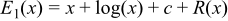#Boost C++ Libraries

...one of the most highly regarded and expertly designed C++ library projects in the world.

This is the documentation for an old version of boost. Click here for the latest Boost documentation.

#### Exponential Integral En

##### Synopsis

```#include <boost/math/special_functions/expint.hpp>
```

```namespace boost{ namespace math{

template <class T>
calculated-result-type expint(unsigned n, T z);

template <class T, class Policy>
calculated-result-type expint(unsigned n, T z, const Policy&);

}} // namespaces
```

The return type of these functions is computed using the result type calculation rules: the return type is `double` if T is an integer type, and T otherwise.

The final Policy argument is optional and can be used to control the behaviour of the function: how it handles errors, what level of precision to use etc. Refer to the policy documentation for more details.

##### Description
```template <class T>
calculated-result-type expint(unsigned n, T z);

template <class T, class Policy>
calculated-result-type expint(unsigned n, T z, const Policy&);
```

Returns the exponential integral En of z:##### Accuracy

The following table shows the peak errors (in units of epsilon) found on various platforms with various floating point types, along with comparisons to the Cephes library. Unless otherwise specified any floating point type that is narrower than the one shown will have effectively zero error.

Table 45. Errors In the Function expint(n, z)

Significand Size

Platform and Compiler

En

E1

53

Win32, Visual C++ 8

Peak=7.1 Mean=1.8

Cephes Peak=5.1 Mean=1.3

Peak=0.99 Mean=0.5

Cephes Peak=3.1 Mean=1.1

64

RedHat Linux IA_EM64, gcc-4.1

Peak=9.9 Mean=2.1

Peak=0.97 Mean=0.4

64

Redhat Linux IA64, gcc-4.1

Peak=9.9 Mean=2.1

Peak=0.97 Mean=0.4

113

HPUX IA64, aCC A.06.06

Peak=23.3 Mean=3.7

Peak=1.6 Mean=0.5

##### Testing

The tests for these functions come in two parts: basic sanity checks use spot values calculated using Mathworld's online evaluator, while accuracy checks use high-precision test values calculated at 1000-bit precision with NTL::RR and this implementation. Note that the generic and type-specific versions of these functions use differing implementations internally, so this gives us reasonably independent test data. Using our test data to test other "known good" implementations also provides an additional sanity check.

##### Implementation

The generic version of this function uses the continued fraction:for large x and the infinite series:for small x.

Where the precision of x is known at compile time and is 113 bits or fewer in precision, then rational approximations devised by JM are used for the ```n == 1``` case.

For ```x < 1``` the approximating form is a minimax approximation:and for ```x > 1``` a Chebyshev interpolated approximation of the form:is used.